Foxtable(狐表)用户栏目专家坐堂 → [求助]第二列前20行的数量自动录入

共有227人关注过本帖树形打印复制链接

# 主题：[求助]第二列前20行的数量自动录入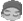miaoqingqing
1楼 | 信息 | 搜索 | 邮箱 | 主页 | UC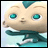加好友发短信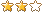[求助]第二列前20行的数量自动录入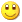Post By：2023/3/10 9:59:00 [只看该作者]

 下载信息  [文件大小：   下载次数： ]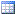点击浏览该文件:第二列前20行数量自动录入到第三列.foxdb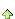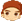2楼 | 信息 | 搜索 | 邮箱 | 主页 | UC加好友发短信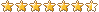Post By：2023/3/10 10:16:00 [只看该作者]

 使用for遍历miaoqingqing
3楼 | 信息 | 搜索 | 邮箱 | 主页 | UC加好友发短信Select Case e.DataCol.Name

Case "第二列","第二列前20行等于4的数量自动录入"

Dim dr As DataRow
Dim drs As List(of DataRow)
dr = e.DataTable.Find("[_SortKey] < " & e.DataRow("_SortKey"), "[_SortKey] Desc") '找出上一行
If dr Is Nothing Then '如果没有找到上一行,说明本行就是第一行
e.DataRow("第二列前20行等于4的数量自动录入") = Nothing
dr = e.DataRow
End If
drs = e.DataTable.Select("[_SortKey] >= " & dr("_SortKey"), "[_SortKey]")

For i As Integer = 20 To drs.Count - 1 '重算余下行的余额
If e.DataRow("第二列") = "4" Then
Dim Products As List(Of String)
Products = DataTables("表A").GetValues("第二列","第二列  = 4")
Dim q As Integer = DataTables("表A").GetValues("第二列","第二列  = 4").Count

'Dim 数组1() As Char = e.DataRow("第二列")
'Dim q As Integer = 数组1.Count

drs(i)("第二列前20行等于4的数量自动录入") = q

End If
Next

End Select
 下载信息  [文件大小：   下载次数： ]点击浏览该文件:狐表不能升级了，代码辛苦贴出来，感谢.foxdb4楼 | 信息 | 搜索 | 邮箱 | 主页 | UC加好友发短信Post By：2023/3/10 13:34:00 [只看该作者]

 Case "第二列","第二列前20行等于4的数量自动录入"                Dim dr As DataRow        Dim drs As List(of DataRow)        dr = e.DataTable.Find("[_SortKey] < " & e.DataRow("_SortKey"), "[_SortKey] Desc") '找出上一行        If dr Is Nothing Then '如果没有找到上一行,说明本行就是第一行            e.DataRow("第二列前20行等于4的数量自动录入") = Nothing            dr = e.DataRow        End If        drs = e.DataTable.Select("[_SortKey] <= " & dr("_SortKey"), "[_SortKey] desc")dim sum as integer            For i As Integer = 0 To math.min(drs.Count - 1,19) '重算余下行的余额If e.DataRow("第二列") = "4" Then sum += 1nexte.DataRow("第二列前20行等于4的数量自动录入") = sum End Selectmiaoqingqing
5楼 | 信息 | 搜索 | 邮箱 | 主页 | UC加好友发短信蓝总，上楼代码 第二列重置列时，项目崩溃报错： 无法创建新的堆x防护页面下面的代码也项目崩溃报错： 无法创建新的堆x防护页面求助Select Case e.DataCol.Name        Case "第二列","第二列前20行等于4的数量自动录入"                Dim dr As DataRow        Dim drs As List(of DataRow)        dr = e.DataTable.Find("[_SortKey] < " & e.DataRow("_SortKey"), "[_SortKey] Desc") '找出上一行        If dr Is Nothing Then '如果没有找到上一行,说明本行就是第一行            e.DataRow("第二列前20行等于4的数量自动录入") = Nothing            dr = e.DataRow        End If        drs = e.DataTable.Select("[_SortKey] <= " & dr("_SortKey"), "[_SortKey] desc")        Dim sum As Integer        For i As Integer = 0 To math.min(drs.Count - 1,19) '重算余下行的余额            If e.DataRow("第二列") = "4" Then                sum += 1                e.DataRow("第二列前20行等于4的数量自动录入") = sum            End If            If e.DataRow("第二列") = "8" Then                sum += 1                e.DataRow("第二列前20行等于8的数量自动录入") = sum            End If        Next        End Select6楼 | 信息 | 搜索 | 邮箱 | 主页 | UC加好友发短信Post By：2023/3/10 15:23:00 [只看该作者]

 Select Case e.DataCol.Name        Case "第二列"                Dim dr As DataRowmiaoqingqing
7楼 | 信息 | 搜索 | 邮箱 | 主页 | UC加好友发短信蓝总，下面的代码生成的数量自动录入结果不对想实现自动录入正确值是从第21行开始统计上面20行含有值的数量，图片粉色打钩处的值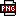此主题相关图片如下：代码生成的值与正切值不符（不是第一列的值）.png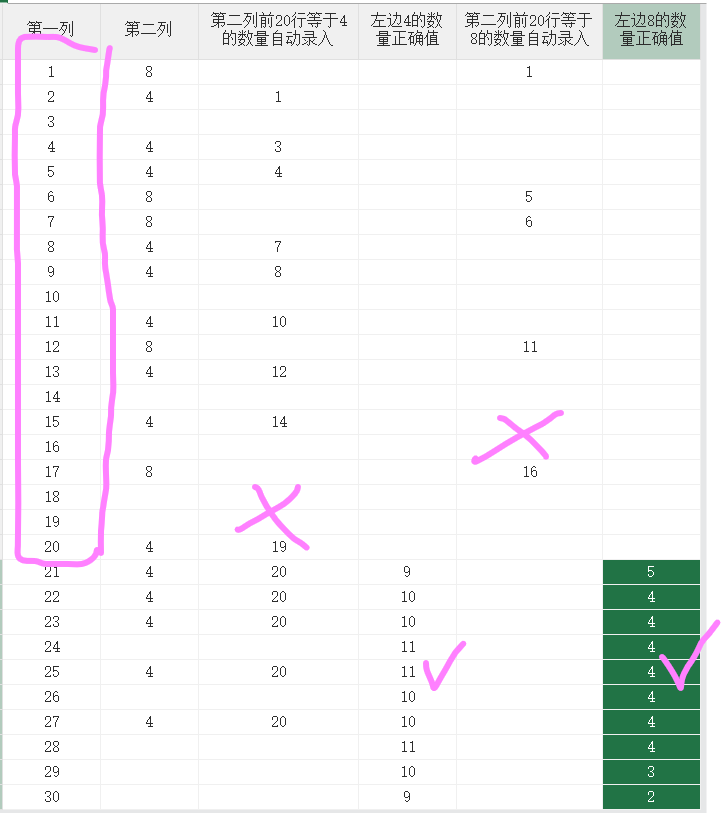Select Case e.DataCol.Name        Case "第二列"                Dim dr As DataRow                Dim drs As List(of DataRow)        dr = e.DataTable.Find("[_SortKey] < " & e.DataRow("_SortKey"), "[_SortKey] Desc") '找出上一行        If dr Is Nothing Then '如果没有找到上一行,说明本行就是第一行            e.DataRow("第二列前20行等于4的数量自动录入") = Nothing            dr = e.DataRow        End If        drs = e.DataTable.Select("[_SortKey] <= " & dr("_SortKey"), "[_SortKey] desc")        Dim sum As Integer        For i As Integer = 0 To math.min(drs.Count - 1,19) '重算余下行的余额            If e.DataRow("第二列") = "4" Then                sum += 1                e.DataRow("第二列前20行等于4的数量自动录入") = sum            End If            If e.DataRow("第二列") = "8" Then                sum += 1                e.DataRow("第二列前20行等于8的数量自动录入") = sum            End If        Next        End Select8楼 | 信息 | 搜索 | 邮箱 | 主页 | UC加好友发短信Post By：2023/3/10 15:53:00 [只看该作者]

 第一列如果是序号，自己根据序号计算不就行了，判断第一列大于20的才才计算。21行就计算序号1~20、22行就计算2~21、...以此类推miaoqingqing
9楼 | 信息 | 搜索 | 邮箱 | 主页 | UC加好友发短信蓝总，第一列不是序号，是无用的列。下面的代码从第21行开始自动录入第二列 值4  值8 的数量还是不对，辛苦帮忙Select Case e.DataCol.Name        Case "第二列"                Dim dr As DataRow                Dim drs As List(of DataRow)        dr = e.DataTable.Find("[_SortKey] < " & e.DataRow("_SortKey"), "[_SortKey] Desc") '找出上一行        If dr Is Nothing Then '如果没有找到上一行,说明本行就是第一行            e.DataRow("第二列前20行等于4的数量自动录入") = Nothing            dr = e.DataRow        End If        drs = e.DataTable.Select("[_SortKey] <= " & dr("_SortKey"), "[_SortKey] desc")        Dim sum As Integer        For i As Integer = 20 To drs.Count - 1 '重算余下行的余额       ' For i As Integer = 20 To math.min(drs.Count - 1,19) '重算余下行的余额            If e.DataRow("第二列") = "4" Then                sum += 1                'e.DataRow("第二列前20行等于4的数量自动录入") = sum                drs(i-20)("第二列前20行等于4的数量自动录入") =  sum            End If            If e.DataRow("第二列") = "8" Then                sum += 1                ' e.DataRow("第二列前20行等于8的数量自动录入") = sum               drs(i-20)("第二列前20行等于8的数量自动录入") =  sum            End If        Next        End Select10楼 | 信息 | 搜索 | 邮箱 | 主页 | UC加好友发短信Post By：2023/3/10 16:37:00 [只看该作者]

 都计算到一起了，肯定不对咯，分开计算。另外计算完毕再给单元格赋值，而不是一边计算一边赋值总数 17 1 2 下一页